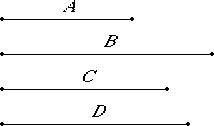# Proposition 115

From a medial straight line there arise irrational straight lines infinite in number, and none of them is the same as any preceding.

Let A be a medial straight line.

I say that from A there arise irrational straight lines infinite in number, and none of them is the same as any of the preceding.Set out a rational straight line B, and let the square on C equal the rectangle B by A. Then C is irrational, for that which is contained by an irrational and a rational straight line is irrational.

And it is not the same with any of the preceding, for the square on none of the preceding, if applied to a rational straight line will produce as breadth a medial straight line.

X.20

Again, let the square on D equal the rectangle B by C. Then the square on D is irrational.

X.Def.I.4

Therefore D is irrational, and it is not the same with any of the preceding, for the square on none of the preceding, if applied to a rational straight line, will produce C as breadth.

Similarly, if this arrangement proceeds ad infinitum, it is manifest that from the medial straight line there arise irrational straight lines infinite in number, and none is the same with any of the preceding.

Q.E.D.

## Guide

This proposition is not used in the rest of the Elements.